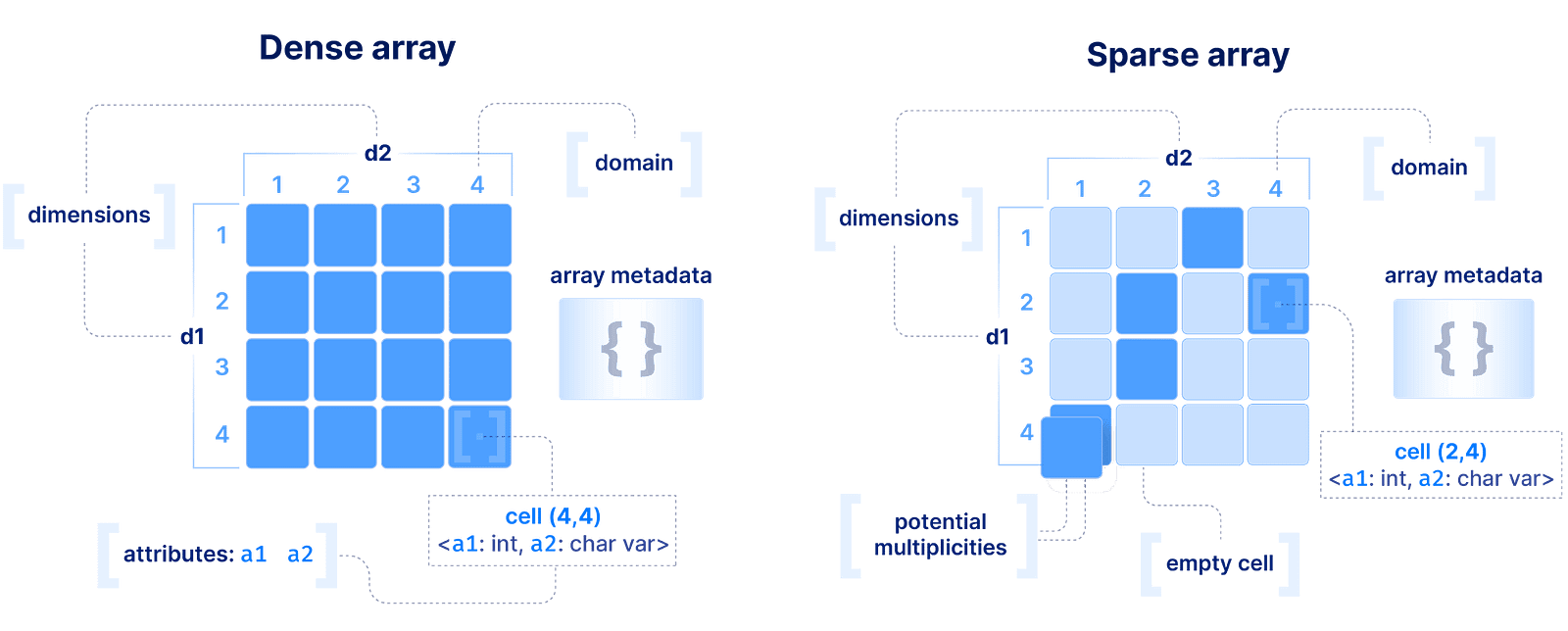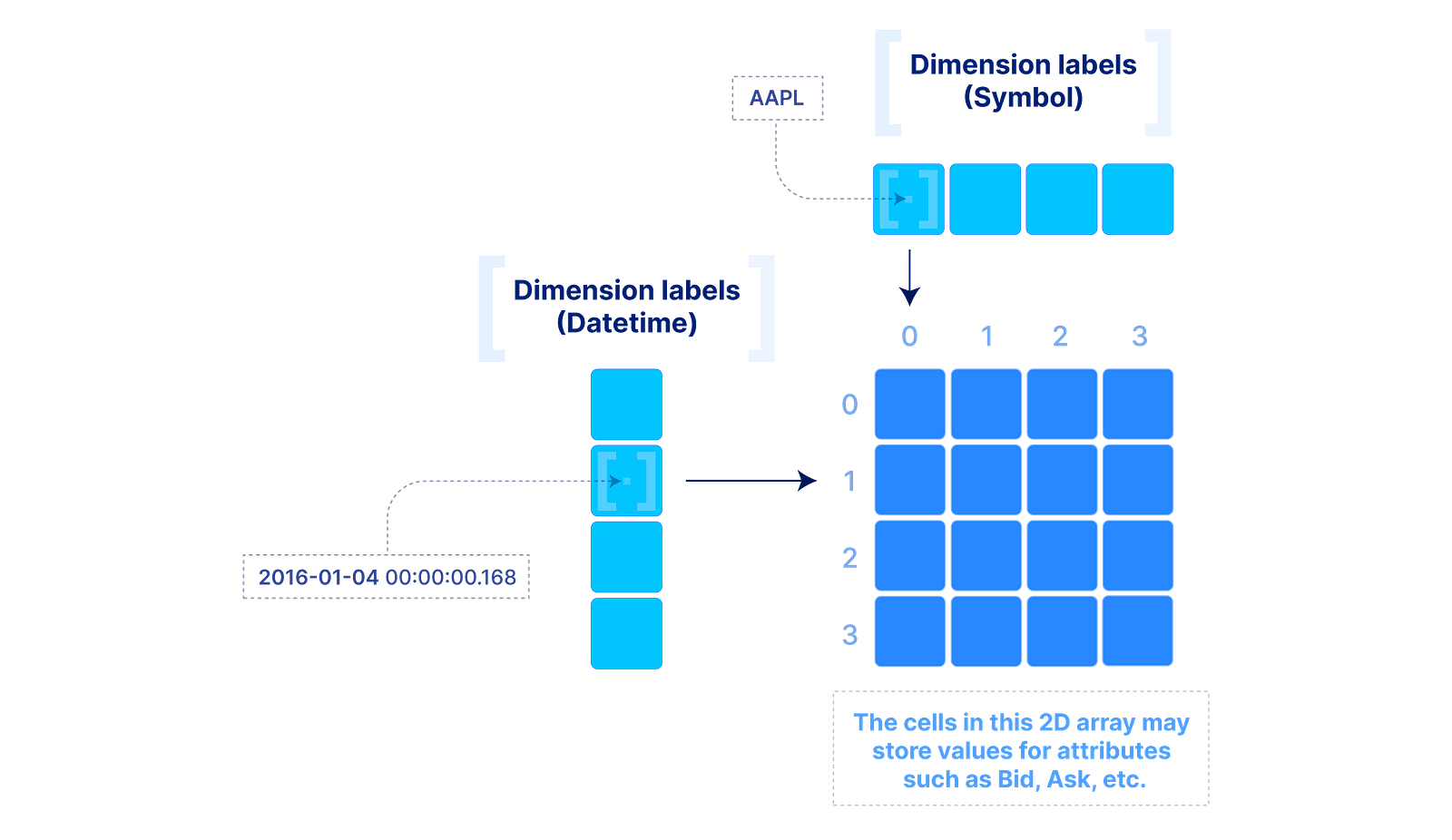# Data Model

## Dense and sparse arrays

The basic array model we follow at TileDB is depicted below. We make an important distinction between a dense and a sparse array. A dense array can have any number of dimensions. Each dimension must have a domain with integer values, and all dimensions have the same data type. An array element is defined by a unique set of dimension coordinates and it is called a cell. In a dense array, all cells must store a value. A logical cell can store more than one value of potentially different types (which can be integers, floats, strings, etc). An attribute defines a collection of values that have the same type across all cells. A dense array may be associated with a set of arbitrary key-value pairs, called array metadata.The array data model
A sparse array is very similar to a dense array, but it has three important differences:
1. 1.
Cells in a sparse array can be empty.
2. 2.
The dimensions can have heterogeneous types, including floats and strings (i.e., the domain can be “infinite”).
3. 3.
Cell multiplicities (i.e., cells with the same dimension coordinates) are allowed.
The decision on whether to model your data with a dense or sparse array depends on the application and it can greatly affect performance. Also extra care should be taken when choosing to model a data field as a dimension or an attribute. These decisions are covered in detail in other sections of the docs, but for now, you should know this: array systems are optimized for rapidly performing range conditions on dimension coordinates. Arrays can also support efficient conditions on attributes, but by design the most optimized selection performance will come from querying on dimensions, and the reason will become clear soon.
Range conditions on dimensions are often called “slicing” and the results constitute a “slice” or “subarray”. Some examples are shown in the figure below. In numpy notation, `A[0:2, 1:3]` is a slice that consists of the values of cells with coordinates 0 and 1 on the first dimension, and coordinates 1 , and 2 on the second dimension (assuming a single attribute). Alternatively, this can be written in SQL as `SELECT attr FROM A WHERE d1>=0 AND d1<=1 AND d2>=1 AND d2<=2` , where `attr` is an attribute and `d1` and `d2` the two dimensions of array `A`. Note also that slicing may contain more than one range per dimension (a multi-range slice/subarray).Slicing array data
The above model can be extended to include “dimension labels”. This extension can be applied to both dense and sparse arrays, but labels are particularly useful in dense arrays. Briefly stated, a dimension can accept a label vector which maps values of arbitrary data types to integer dimension coordinates. An example is demonstrated below. This is very powerful in applications where the data is quite dense (i.e., there are not too many empty cells), but the dimension fields are not integers or do not appear contiguously in the integer domain. In such cases, multi-dimensional slicing is performed by first efficiently looking up the integer coordinates in the label vectors, and then applying the slicing as explained above, which in the dense array case can be truly rapid.Dense array with labeled dimensions
Labeled dimensions are currently under development in TileDB. They will appear in a future release soon.

## Groups

In many applications, it is useful to hierarchically organize different arrays into groups. TileDB incorporates the concept and functionality of groups into its Data Format.